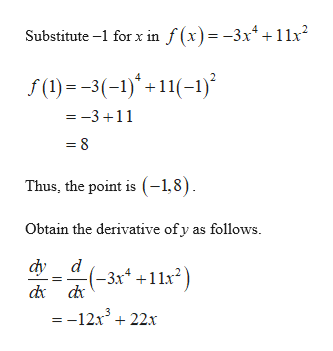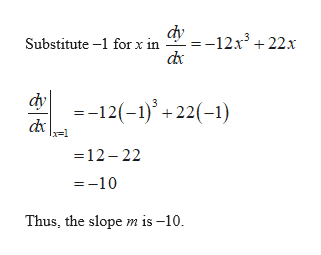# f(x)=-3x^4+11x^2What is the equation of the tangent line at x=-1?

Question
1 views

f(x)=-3x^4+11x^2

What is the equation of the tangent line at x=-1?

check_circle

Step 1

Obtain the value of y as follows.help_outlineImage TranscriptioncloseSubstitute - for x in / (x)=-3x4+11x2 f(1)3(-)11-) =-3 11 8 Thus, the point is (-1,8) Obtain the derivative of y as follows. фу d -3x+11x2 dxdx =-12x3 22x fullscreen
Step 2

Obtain the slope m as...help_outlineImage TranscriptioncloseSubstitute -1 for x in =-12x3+22:x dx =-12(-1)22(-1) =12-22 =-10 Thus, the slopem is -10 fullscreen

### Want to see the full answer?

See Solution

#### Want to see this answer and more?

Solutions are written by subject experts who are available 24/7. Questions are typically answered within 1 hour.*

See Solution
*Response times may vary by subject and question.
Tagged in

### Other